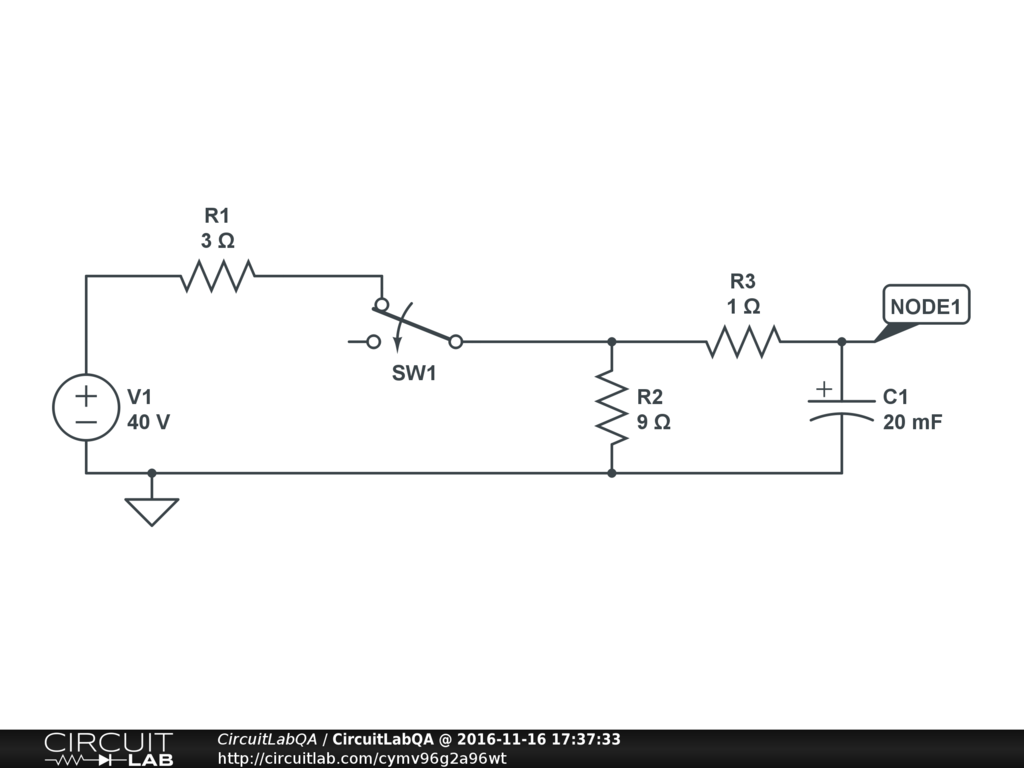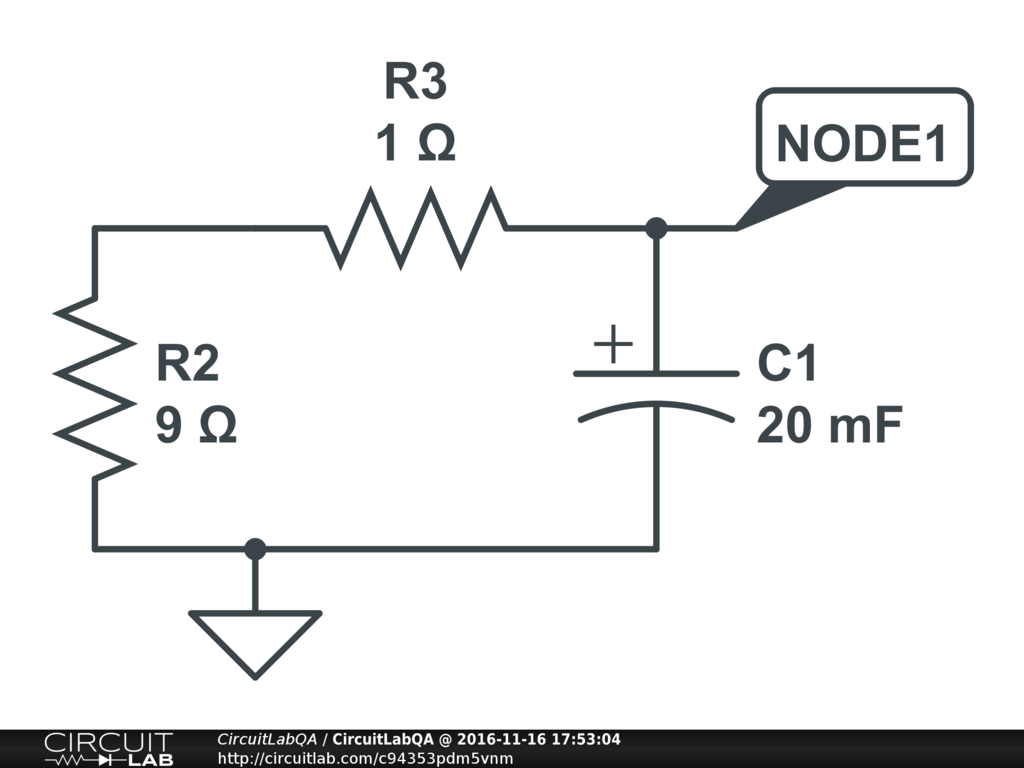## Voltage drop across capacitor in series with resistor

 OK this is a homework problem:For $t < 0$ the switch is closed, but it opens at $t = 0$. Write an equation for $V_{NODE1}(t)$ for $t \ge 0$. Calculate the initial energy stored in the capacitor. I'm trying to figure out the initial voltage of the capacitor. The textbook answer says it's using voltage divider formula, $V_1 \times \frac {R_2} {R_1 + R_2} = 40 \times \frac {9} {9 + 3} = 30$. That's what the simulator says, $V_{NODE1} = 30$ at $t=0$. But I don't understand. Why is the initial voltage at the capacitor also 30 volts? It has the 1 ohm resistor in series with the source, so surely there must be a voltage drop across that, so less voltage across the capacitor, right? by carrolllip86 November 16, 2016

 When using such ginormous capacitors be sure to leave the vent plug unobstructed. On one product our designers had failed to do this and there were holes in the roof where the caps had gone ballistic, fortunately missing our test guys in transit. +2 votes by mikerogerswsm November 21, 2016

 No. At steady state there's no current through the 1 ohm resistor, so there's no voltage drop. so the capacitor voltage is the same as the R1/R2 voltage divider. +1 vote by blairem November 16, 2016

 @blairem is spot on. Another approach..... at steady state $$\frac{d}{dt}=0$$ For any capacitor $$Q = C \times V$$ and $$i = \frac {d} {dt} Q$$ (that's just how current is defined into a capacitor, it's the rate of change of charge flowing in or out). So since $$\frac {d} {dt} Q = 0$$ that means $$i=0$$ into any capacitor at steady state. Now you look at your schematic, and the R3 and C1 are in series, so you write Kirchoff's Current Law at that node, $$i_R3 - i_C1 = 0$$ or $$i_R3 = i_C1$$ or $$i_R3 = 0$$ so since for a resistor R3 it's $$V_{across R3} = R_3 \times i_R3$$ that means $$V_{across R3} = 0$$ too. Too many equations just to say ZERO. +1 vote by SJohnsson November 16, 2016

 Question 1: Initial voltage is 30. Time constant is just C1 discharging through R2+R3 in series, because this is what the circuit looks like when the switch opens:so $\tau = (20mF)*(9+1 \Omega) = 0.2 seconds$. Exponential decay: $$V_{NODE1}(t) = 30 e^{-t/{0.2 seconds}}$$ Question 2: Initial voltage is 30. Capacitor energy stored is just $$E = \frac{1}{2} C V^2$$. so $$E=\frac{1}{2} 20mF \times (30 V)^2 = 9 Joules$$ Thats a big capacitor!!! +1 vote by gavers7 November 16, 2016

CircuitLab's Q&A site is a FREE questions and answers forum for electronics and electrical engineering students, hobbyists, and professionals.

We encourage you to use our built-in schematic & simulation software to add more detail to your questions and answers.

Acceptable Questions:

• Concept or theory questions
• Practical engineering questions
• “Homework” questions
• Software/hardware intersection
• Best practices
• Design choices & component selection
• Troubleshooting

Unacceptable Questions:

• Non-English language content
• Non-question discussion
• Non-electronics questions
• Vendor-specific topics
• Pure software questions
• CircuitLab software support

Please respect that there are both seasoned experts and total newbies here: please be nice, be constructive, and be specific!